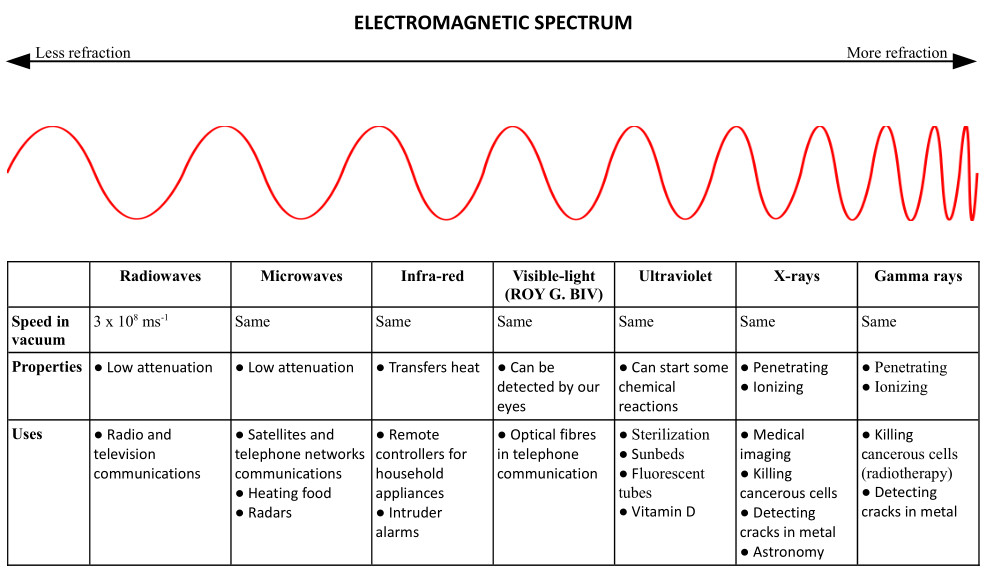Home » Handbook of O Level Physics » UNIT 13: GENERAL WAVE PROPERTIES

# UNIT 13: GENERAL WAVE PROPERTIES

• Wave motion

• It is the transfer of energy

• without (net) transfer of material medium

• Types of waves

• Transverse waves: These are waves in which the direction of wave-motion is perpendicular to the direction of vibration of source.

• Examples: Rope waves, all kinds of electromagnetic radiations, water waves, etc.

• longitudinal waves: These are waves in which the direction of wave-motion is parallel to the direction of vibration of source.

• Examples: Sound waves, compressions and rarefactions in a slinky spring, etc.

• Some terminologies related to the study of wave-motion are:

• Crests: They are the high points. For longitudinal waves the term compressions is used.

• Troughs: They are the low points. For longitudinal waves the term rarefactions is used.

• Amplitude (A): It is the MAXIMUM displacement from the central position.

• Wavelength (λ): It is the displacement between two neighbouring crests.

• Frequency (f): It is the number of waves per unit time.

• Unit: Hz

• Period (T): It is the time taken to produce one complete wave.

• Wavefront: It is the imaginary line on a wave that joins all the crests. Wavefronts can be

• circular wavefronts

• plane wavefronts

• Formulae / Relationships

f = 1 / T

v = f λ

• A ripple tank can help us study waves including

• reflection

• refraction

• Remember “SSS” – Shallower Shorter Slower.

• Frequency of a wave cannot change because it depends on source.

• Electromagnetic spectrum

• Different range of wavelength have been given different names, e.g., radio waves, infrared, etc.

• They are all transverse waves.

• They travel at 3,00,000,000 m/s in vacuum.

• They slow down in other mediums.

• They carry no charge.

• Fluorescence: It is the visible light emitted during absorption of Ultraviolet, X-rays or Gamma rays.